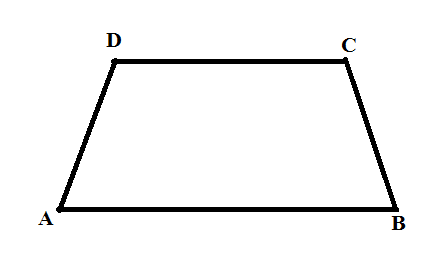Home/Class 8/Maths/

The angles of a quadrilateral ABCD taken in an order are in the ratio $$3:7:6:4$$ . Then $$ABCD$$ is a （ ）
A. kite
B. parallelogram
C. rhombus
D. trapeziumSpeed
00:00
07:12## QuestionMathsClass 8

The angles of a quadrilateral ABCD taken in an order are in the ratio $$3:7:6:4$$ . Then $$ABCD$$ is a （ ）
A. kite
B. parallelogram
C. rhombus
D. trapezium

D
4.64.6## Solution

Let the angles of quadrilateral $$ABCD$$ be $$\angle A=3x,\angle B=7x,\angle C=6x,\angle D=4x$$
We know that sum of all angles of a quadrilateral $$=360^{\circ}$$ .
$$\Rightarrow 3x+7x+6x+4x=360^{\circ}$$.
$$\Rightarrow 20x=360^{\circ}$$
$$x= \frac{360^{\circ}}{20} = 18^{\circ}$$.
Hence, $$\angle A=3x=3\times 18^{\circ}=54^{\circ}$$, $$\angle B = 7\times 18^{\circ}= 126^{\circ}$$, $$\angle C=6x=6\times 18^{\circ}= 108^{\circ}$$, $$\angle D = 4x=4\times 18^{\circ}=72^{\circ}$$.
$$\angle A + \angle B = 54^{\circ} +126^{\circ} =180^{\circ}$$ (Satisfying the corresponding angles)...($$1$$)
$$\angle C +\angle D = 108^{\circ}+72^{\circ}=180^{\circ}$$ (Satisfying the corresponding angles)...($$2$$)
From $$(1)$$ and $$(2)$$
Since the corresponding angles property is satisfies. Hence, $$AB\parallel CD$$.
Hence, the given figure is a trapezium.